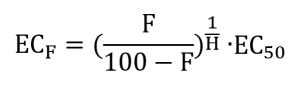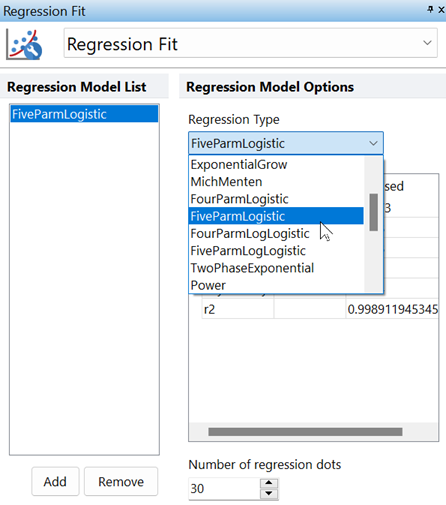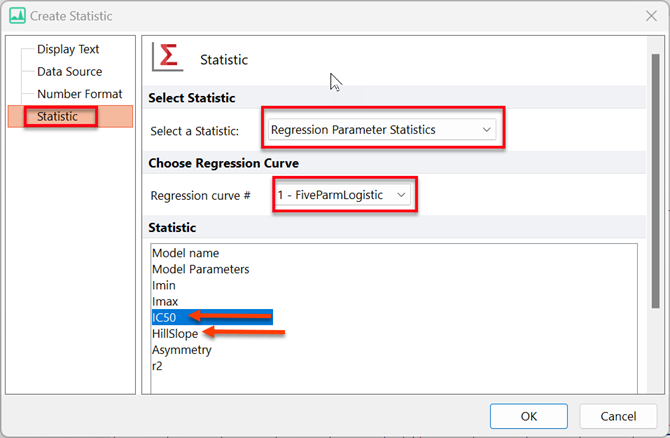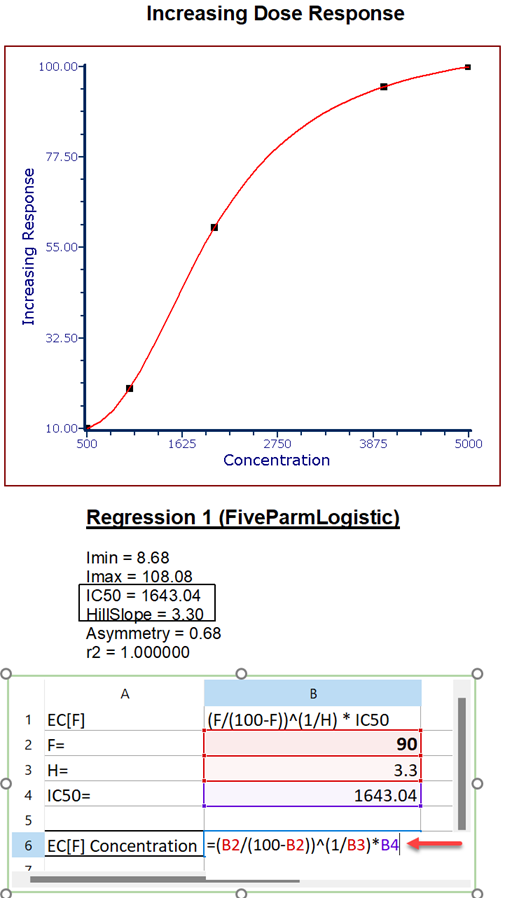# How do I calculate EC/IC Anything?

What is EC/IC Anything?

The potency of a drug is commonly quantified as the EC50, the concentration that leads to 50% maximal response.  But in some systems you might be more interested in the EC80 or the EC90 or some other value. You can compute these values from the EC50 to provide ECanything.

If your response goes down with increasing dose (so the Hillslope is negative), watch out for the terminology. The EC10 (for example) is defined as the concentration that gives you a response 10% of the way between the Bottom and Top plateaus. In situations where the response goes down as you increase dose, you may think of this as the "IC90" since it gives 90% of the maximum inhibition.

Calculating EC/IC Anything from the EC50

The calculation for the EC/IC anything is deriving the value from the EC50 using the following formula, where F is the value you wish to set:Let's say you set F=80. Then the ECF for inhibitory data would be the concentration (X value) required to bring the curve down to 80%. If you actually want the concentration that brings the curve down by 80%, to 20%, then you'd need to set F equal to 20 in the formula above.

We have summarized these ideas in the following table for your future reference:

 Correlation The value to report Value to plug into the formula Response increases as concentration increases (effective response) ECF F Response decreases as concentration increases (inhibitive response) ICF 100-F

Please see the source materials and a further discussion of EC/IC anything calculations on the GraphPad Prism website

How to Calculate EC/IC Anything in FCS Express

With FCS Express you can easily calculate your own value for EC or IC. View our example EC/IC anything calculation in our example layout. You may download the example layout here.

EC/IC Anything is calculated within FCS Express using regression plots. We can take certain variables as calculated from the best-fit curve. We then plug the variables into a formula within our internal spreadsheet.

Step by Step Considerations

• The first step is to ensure that your data is represented within a spreadsheet in FCS Express. You will require two columns, one for concentration and one for a read out of response.
• From here, you can create a scatter with regression plot. This will need to be formatted to a FiveParmLogistic (Five Parameter Logistic) regression Model.• Two statistics are required from this plot (the IC50 and the Hillslope).
Note: EC50 and IC50 are equal, within FCS Express, the statistic is named "IC50", which can be used for both values.

• One way to report these statistics is by right-clicking on the Scatter with Regression plot > Statistics > Regression Parameter Statistics
• These statistics can be directly reported in an integrated spreadsheet by:
• Right-clicking inside a Spreadsheet Cell >Insert Token > Statistic
• Selecting the correct regression plot to source the statistics from.• Then, in the Statistic menu, selecting the Regression Parameter Statistics from the drop down menu.• In addition to these statistics, we recommend entering the F value into the spreadsheet.
• These three values, the IC50, the Hillslope and the F value, can be plugged into a formula to retrieve your desired EC/IC anything value.If you would like multiple EC/ICs it is simply a matter of creating additional cells utilising the same formula.